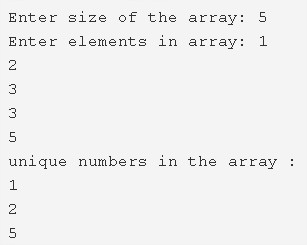# C Program To Print All Unique Elements In The Array | C Programs

C Program to print all unique elements in the array – In this article, we will discuss the various ways to print all the unique elements in the array in C programming.

Suitable examples and sample programs have also been added so that you can understand the whole thing very clearly. The compiler has also been added with which you can execute it yourself.

The ways described in this piece are as follows:

• Using Standard Method
• Using Function

As we all know, arrays are a sequential arrangement of a bunch of elements in a horizontal fashion. Arrays form an important part of C programming. The elements of an array are denoted with the help of pointers.As we can see in the image given above, firstly, we have to enter the size of the array.

The size of the array in the above image is 5.

Then, you need to enter the elements specified for the particular array.

The elements in the array given above are as follows:

1 2 3 3 5

As you can see, the element 3 is repeated. It is not unique in nature compared to the other elements of the array.

So, the unique numbers in the array are as follows:

1 2 5

Hence, the multiple means to find out the same in C programming are as follows:

## Using Standard Method

1. Read and store the entered array size into the variable n.

2) Read the elements and store into the array a[] as scanf(“%d”,&a[i]) using for loop for(i=0;i<n;i++).

3) Find the repeated elements in the array

for loop iterates from i=0 i<n,c=1

a) If the a[i]!=-1 then compare each element with remaining elements of the array as a[i]==a[j],using for loop from j=i+1 to j<n.

If any element is equal to other elements of the array then increase the c value by 1.Repeat the comparison until j<n.

b) Store the c value into b[i].Repeat until i<n. The array b[] contains the count values of the array elements.

4) The count value represents how many times the element repeated in the array. The count 1 means the element repeated only once in the array which is unique.

Print the unique elements which are having the count value is 1 using for loop from i=0 to i<n.

Output:

### Using Function

1. The main() calls the count() function by passing a,b,n are arguments to the function.

2) The count() function finds the count values of each element of the array where count represents how many times each element repeated in the array.

for loop iterates from i=0 to i<n, count c=1,

a) If a[i]!=-1 then, compare each element with remaining elements of the array and find how many times each element repeated in the array i.e count using for loop for(j=i+1;j<n;j++).

b) Store the count value into the array b[], repeat until i<n.

3) Print the unique elements which is having the count value 1 using the print function as

If b[i]==1 then print a[i] using for loop for(i=0;i<n;i++).

Output:

x

## C Program Inverted Pyramid Star Pattern | 4 Ways – C Programs

C Program to print an inverted pyramid star pattern – In this article, we will ...